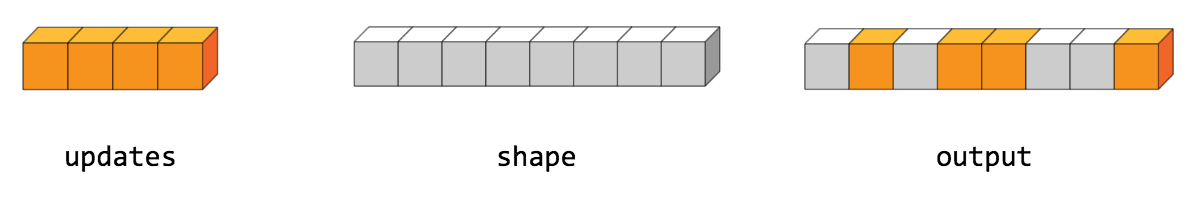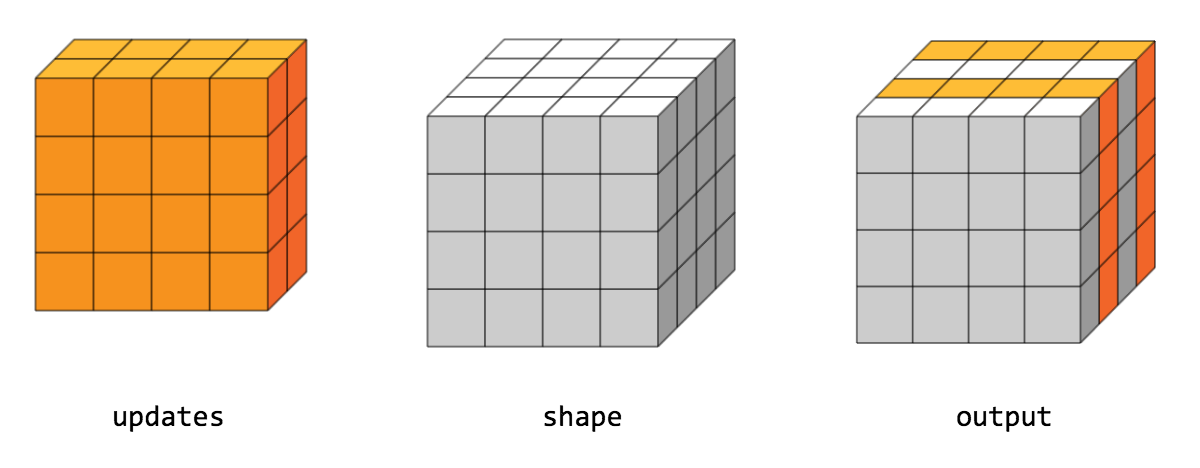# ScatterNd

public final class ScatterNd

Scatter `updates` into a new tensor according to `indices`.

Creates a new tensor by applying sparse `updates` to individual values or slices within a tensor (initially zero for numeric, empty for string) of the given `shape` according to indices. This operator is the inverse of the `tf.gather_nd` operator which extracts values or slices from a given tensor.

This operation is similar to tensor_scatter_add, except that the tensor is zero-initialized. Calling `tf.scatter_nd(indices, values, shape)` is identical to `tensor_scatter_add(tf.zeros(shape, values.dtype), indices, values)`

If `indices` contains duplicates, then their updates are accumulated (summed).

WARNING : The order in which updates are applied is nondeterministic, so the output will be nondeterministic if `indices` contains duplicates -- because of some numerical approximation issues, numbers summed in different order may yield different results.

`indices` is an integer tensor containing indices into a new tensor of shape `shape`. The last dimension of `indices` can be at most the rank of `shape`:

indices.shape[-1] <= shape.rank

The last dimension of `indices` corresponds to indices into elements (if `indices.shape[-1] = shape.rank`) or slices (if `indices.shape[-1] < shape.rank`) along dimension `indices.shape[-1]` of `shape`. `updates` is a tensor with shape

indices.shape[:-1] + shape[indices.shape[-1]:]

The simplest form of scatter is to insert individual elements in a tensor by index. For example, say we want to insert 4 scattered elements in a rank-1 tensor with 8 elements.In Python, this scatter operation would look like this:

``````indices = tf.constant([, , , ])
updates = tf.constant([9, 10, 11, 12])
shape = tf.constant()
print(scatter)
``````
The resulting tensor would look like this:

[0, 11, 0, 10, 9, 0, 0, 12]

We can also, insert entire slices of a higher rank tensor all at once. For example, if we wanted to insert two slices in the first dimension of a rank-3 tensor with two matrices of new values.In Python, this scatter operation would look like this:

``````indices = tf.constant([, ])
updates = tf.constant([[[5, 5, 5, 5], [6, 6, 6, 6],
[7, 7, 7, 7], [8, 8, 8, 8]],
[[5, 5, 5, 5], [6, 6, 6, 6],
[7, 7, 7, 7], [8, 8, 8, 8]]])
shape = tf.constant([4, 4, 4])
print(scatter)
``````
The resulting tensor would look like this:

[[[5, 5, 5, 5], [6, 6, 6, 6], [7, 7, 7, 7], [8, 8, 8, 8]], [[0, 0, 0, 0], [0, 0, 0, 0], [0, 0, 0, 0], [0, 0, 0, 0]], [[5, 5, 5, 5], [6, 6, 6, 6], [7, 7, 7, 7], [8, 8, 8, 8]], [[0, 0, 0, 0], [0, 0, 0, 0], [0, 0, 0, 0], [0, 0, 0, 0]]]

Note that on CPU, if an out of bound index is found, an error is returned. On GPU, if an out of bound index is found, the index is ignored.

### Public Methods

 Output () Returns the symbolic handle of a tensor. static ScatterNd ( Scope scope, Operand indices, Operand updates, Operand shape) Factory method to create a class wrapping a new ScatterNd operation. Output () A new tensor with the given shape and updates applied according to the indices.

## Public Methods

#### public Output <U> asOutput ()

Returns the symbolic handle of a tensor.

Inputs to TensorFlow operations are outputs of another TensorFlow operation. This method is used to obtain a symbolic handle that represents the computation of the input.

#### public static ScatterNd <U> create ( Scope scope, Operand <T> indices, Operand <U> updates, Operand <T> shape)

Factory method to create a class wrapping a new ScatterNd operation.

##### Parameters
 scope current scope Index tensor. Updates to scatter into output. 1-D. The shape of the resulting tensor.
##### Returns
• a new instance of ScatterNd

#### public Output <U> output ()

A new tensor with the given shape and updates applied according to the indices.

[]
[]